Test: Context-Free Grammars & Push-Down Automata

# Test: Context-Free Grammars & Push-Down Automata

Test Description

## 30 Questions MCQ Test GATE Computer Science Engineering(CSE) 2023 Mock Test Series | Test: Context-Free Grammars & Push-Down Automata

Test: Context-Free Grammars & Push-Down Automata for Computer Science Engineering (CSE) 2023 is part of GATE Computer Science Engineering(CSE) 2023 Mock Test Series preparation. The Test: Context-Free Grammars & Push-Down Automata questions and answers have been prepared according to the Computer Science Engineering (CSE) exam syllabus.The Test: Context-Free Grammars & Push-Down Automata MCQs are made for Computer Science Engineering (CSE) 2023 Exam. Find important definitions, questions, notes, meanings, examples, exercises, MCQs and online tests for Test: Context-Free Grammars & Push-Down Automata below.
Solutions of Test: Context-Free Grammars & Push-Down Automata questions in English are available as part of our GATE Computer Science Engineering(CSE) 2023 Mock Test Series for Computer Science Engineering (CSE) & Test: Context-Free Grammars & Push-Down Automata solutions in Hindi for GATE Computer Science Engineering(CSE) 2023 Mock Test Series course. Download more important topics, notes, lectures and mock test series for Computer Science Engineering (CSE) Exam by signing up for free. Attempt Test: Context-Free Grammars & Push-Down Automata | 30 questions in 90 minutes | Mock test for Computer Science Engineering (CSE) preparation | Free important questions MCQ to study GATE Computer Science Engineering(CSE) 2023 Mock Test Series for Computer Science Engineering (CSE) Exam | Download free PDF with solutions
 1 Crore+ students have signed up on EduRev. Have you?
Test: Context-Free Grammars & Push-Down Automata - Question 1

### Consider the following languages.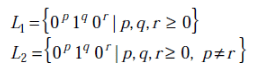Q. Which one of the following statements is FALSE?

Detailed Solution for Test: Context-Free Grammars & Push-Down Automata - Question 1

(D) is false. L1 is regular, so its complement would also be regular. L1 is a regular language of the form 0^* 1^* 0^*. L2 on the other hand is a CFL as it can be derived from the following CFG L2 = {0^p 1^q 0^r | p,q,r>0   And p notEqualTo r} S -> AC|CA C -> 0C0|B A -> 0A|0 B -> 1B|epsilon If coming up with a CFG for L2 is difficult, one can intuitively see that by reducing it to a simpler problem. L2 is very similar to a known CFL L3 = {a^m b^l | m notEqualTo n}
(A) L2 is context free, which is true [CORRECT]
(B) L1 intersection L2 is context free, which is again true because L1 is a regular language and L2 is a CFL. RL union CFL is always a CFL. Hence [CORRECT]
(C) Complement of L2 is recursive, which is true due to the fact that complement of a CFL is CSL for sure (Context sensitive language), which in turn (CSL) is a subset of recursive languages. Hence [CORRECT]
(D) Complement of L1 is context free but not regular, which is false due to closure laws of regular languages. Complement of a RL is always a RL. Hence [INCORRECT]

Test: Context-Free Grammars & Push-Down Automata - Question 2

### Which of the following pairs have DIFFERENT expressive power?

Detailed Solution for Test: Context-Free Grammars & Push-Down Automata - Question 2

NDPDA can handle languages or grammars with ambiguity, but DPDA cannot handle languages with ambiguity and any context-free grammar.

Test: Context-Free Grammars & Push-Down Automata - Question 3

### Let P be a regular language and Q be context-free language such that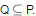(For example, let P be the language represented by the regular expression p*q* and Q be {pnqn|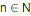}). Then which of the following is ALWAYS regular?

Detailed Solution for Test: Context-Free Grammars & Push-Down Automata - Question 3

1. P ∩ Q would be Q, due to the given fact that Q ⊆ P, hence context free but not regular.
2. P − Q = P ∩ Q might not even be a context free language, due to the closure properties of context free languages.
3. Σ∗ − P is equivalently complement of P, hence regular. Refer to closure laws of regular languages.
4. Σ∗ − Q is equivalently complement of Q, hence it might not even be a context free language.

Test: Context-Free Grammars & Push-Down Automata - Question 4

Consider the language L1,L2,L3 as given below.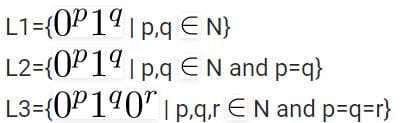Which of the following statements is NOT TRUE?

Detailed Solution for Test: Context-Free Grammars & Push-Down Automata - Question 4

L1 is regular. Its DFA is given as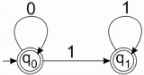L2 is not regular, can be proved using pumping lemma (refer to Ullman). But L2 is CFL.
S → AB
A → 0A|ε
B → 1B|ε

L3 is not CFL, can be proved using pumping lemma (refer to Ullman). But L3 is Recursive.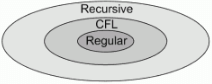Every regular language is also a CFL. So PDA can be used to recognized L1 and L2. As a CFL and Regular language is algo a Recursive language. Hence, Turing machine can be used to recognise L1, L2 and L3. L2 is not regular, can be proved using pumping lemma (refer to Ullman). But L2 is CFL.
S → AB
A → 0A|ε
B → 1B|ε

L3 is not CFL, can be proved using pumping lemma (refer to Ullman). But L3 is Recursive. Every regular language is also a CFL. So PDA can be used to recognised L1 and L2. As a CFL and Regular language is algo a Recursive language. Hence, turing machine can be used to recognise L1, L2 and L3.

Test: Context-Free Grammars & Push-Down Automata - Question 5

Consider the languages L1 = {0i1j | i != j}. L2 = {0i1j | i = j}. L3 = {0i1j | i = 2j+1}. L4 = {0i1j | i != 2j}.

Detailed Solution for Test: Context-Free Grammars & Push-Down Automata - Question 5

All these languages have valid CFGs that can derive them. Hence, all of them are CFLs. Intuitively, (A) & (B) are well known CFLs and CFGs for (C) & (D) could be made by little modifications in A & B’s CFGs.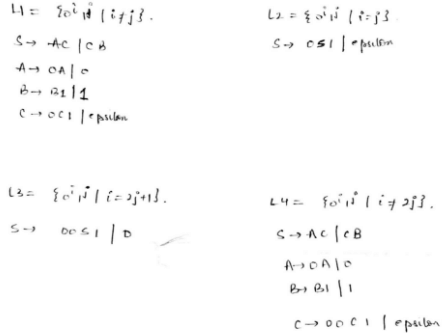Test: Context-Free Grammars & Push-Down Automata - Question 6

S -> aSa|bSb|a|b; The language generated by the above grammar over the alphabet {a,b} is the set of

Detailed Solution for Test: Context-Free Grammars & Push-Down Automata - Question 6

The possible palindrome generated by above grammar can be of odd length only as there is no rule for S -> [Tex]epsilon[/Tex] For example generated palindromes are aba, aaa, bab, ababa, aaaaa, ..

Test: Context-Free Grammars & Push-Down Automata - Question 7

Let L = L1 ∩ L2, where L1 and L2 are languages as defined below:

L1 = {ambmcanbn | m, n >= 0} L2 = {aibjck | i, j, k >= 0}

Then L is

Detailed Solution for Test: Context-Free Grammars & Push-Down Automata - Question 7

The language L1 accept strings {c, abc, abcab, aabbcab, aabbcaabb, …} and L2 accept strings {a, b, c, ab, abc, aabc, aabbc, … }. Intersection of these two languages is L1 cap L2 = {a^{k}b^{k}c | k >= 0} which is context free, but not regular.

Test: Context-Free Grammars & Push-Down Automata - Question 8

The language L= {0i21i | i≥0 } over the alphabet {0,1, 2} is:

Detailed Solution for Test: Context-Free Grammars & Push-Down Automata - Question 8

Let us first design a deterministic pushdown automata for the given language.

• For each occurrence of ‘0’ , we PUSH X in the stack.
• When ‘2’ appears, no stack operation is performed. But, state of the automata is changed.
• For each occurrence of ‘1’ , we POP X from the stack.
• If at the end Z0 is on the stack top then input string is accepted

We also design a Turing machine for the given language.

• When ‘0’ appears in the input string , we replace it with X .Then, traverse to the rightmost corner and replace ‘1’ with Y.
• We go back to the leftmost ‘0’ and repeat the above process.
• While traversing rightwards from the beginning of the input string, if after X, ‘2’ appears and after ‘2’, Y appears then we reach the HALT state. Thus, the given language is recursive. Every recursive language is a CFL. Thus, option (B) is the answer. Please comment below if you find anything wrong in the above post.
Test: Context-Free Grammars & Push-Down Automata - Question 9

Consider the CFG with {S,A,B) as the non-terminal alphabet, {a,b) as the terminal alphabet, S as the start symbol and the following set of production rules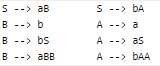Q. Which of the following strings is generated by the grammar?

Detailed Solution for Test: Context-Free Grammars & Push-Down Automata - Question 9

Given below production rules.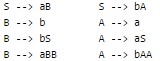We can derive aabbab using below sequence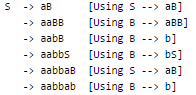Test: Context-Free Grammars & Push-Down Automata - Question 10

For the correct answer strings to above question, how many derivation trees are there?

Detailed Solution for Test: Context-Free Grammars & Push-Down Automata - Question 10

When it asks about the no of derivations tree, we should consider either left most derivation(LMD) or right most derivations(RMD), but not both. Here two left most derivations are possible for the correct string of the previous question "aabbab" from the given grammar. LMD-1 S -> aB [Using S --> aB] -> aaBB [Using B --> aBB] -> aabB [Using B --> b] -> aabbS [Using B --> bS] -> aabbaB [Using S --> aB] -> aabbab [Using B --> b] LMD-2 S -> aB [Using S --> aB] -> aaBB [Using B --> aBB] -> aabSB [Using B --> bS] -> aabbAB [Using S --> bA] -> aabbaB [Using A --> a] -> aabbab [Using B --> b] The Derivation tress are shown below :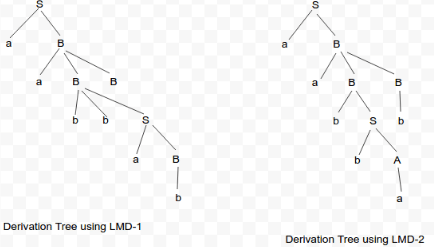Test: Context-Free Grammars & Push-Down Automata - Question 11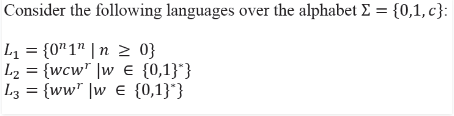Here, wr is the reverse of the string w. Which of these languages are deterministic Context-free languages?

Test: Context-Free Grammars & Push-Down Automata - Question 12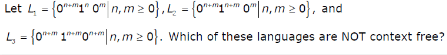Detailed Solution for Test: Context-Free Grammars & Push-Down Automata - Question 12

A PDA can be built only for L1. It is not possible to build PDA for L2 and L3.

Test: Context-Free Grammars & Push-Down Automata - Question 13

Consider the following statements about the context free grammar

G = {S → SS, S → ab, S → ba, S → Ε}
I. G is ambiguous
II. G produces all strings with equal number of a’s and b’s
III. G can be accepted by a deterministic PDA.

Q. Which combination below expresses all the true statements about G?

Detailed Solution for Test: Context-Free Grammars & Push-Down Automata - Question 13

Statement I: G is ambiguous because, as shown in the image below there can be two decision tree for string S = ababab [TRUE]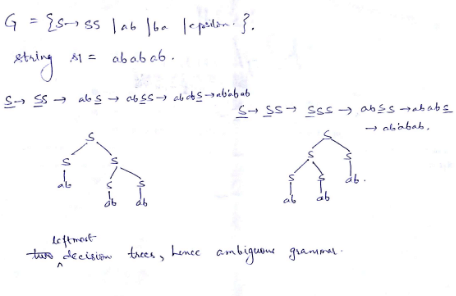Statement II: G produces all strings with equal number of a’s and b’s [FALSE] string 'aabb' cannot be produced by G
Statement III: G can be accepted by a deterministic PDA [TRUE] Assume there is a PDA which pushes if top of the stack is \$ (bottom most alphabet of the stack) and pops otherwise. A string is rejected while popping if the current letter and top of the stack are same. This PDA can derive G. Hence, correct answer should be (B) I and III only Reference: Ambiguity in Context free Grammar and Context free Languages

Test: Context-Free Grammars & Push-Down Automata - Question 14

Which one of the following grammars generates the language L = {aibj | i ≠ j}

Detailed Solution for Test: Context-Free Grammars & Push-Down Automata - Question 14

Language L contains the strings : {abb, aab, abbb, aabbb, aaabb, aa, bb, .......}, i.e, all a's appear before b's in a string, and "number of a's" is not equal to "number of b's", So i ≠ j.

Here Grammar A, B & C also generate the string "ab", where i = j, and many more strings with i = j, hence these grammars do not generate the language L, because for a string that belongs to language L, exponent i should not be equal to exponent  j.

Grammar D : This Grammar never generates a string with equal no of a's and b's, i.e. i=j. Hence this grammar generates the language L. Hence Option D.

Test: Context-Free Grammars & Push-Down Automata - Question 15

In the correct grammar of above question, what is the length of the derivation (number of steps starring from S) to generate the string albm with l ≠ m?

Detailed Solution for Test: Context-Free Grammars & Push-Down Automata - Question 15

Correct grammar of the last question was (D), which is: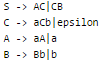Now, the most optimal and intuitive way to generate a string of the form albm would be to first use "C -> aCb|epsilon" production rule to get as many a and b as we can, which would be min(l,m). To get the rest of the string, we could just use latter two production rules accordingly. Formally deriving the string of the general format albm from the above grammar -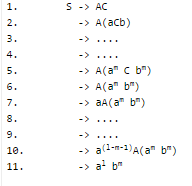From above set of derivation steps we can count the total steps as follows: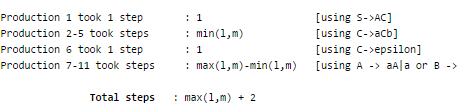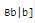Hence, answer should be (A): max(l,m) + 2

Test: Context-Free Grammars & Push-Down Automata - Question 16

Consider the languages:

L1 = {anbncm | n, m > 0} L2 = {anbmcm | n, m > 0}

Q. Which one of the following statements is FALSE?

Detailed Solution for Test: Context-Free Grammars & Push-Down Automata - Question 16

L1 and L2 are context free languages. See this for closure properties.

Test: Context-Free Grammars & Push-Down Automata - Question 17

Consider the languages:

L1 = {wwR |w ∈ {0, 1}*}
L2 = {w#wR | w ∈ {0, 1}*}, where # is a special symbol
L3 = {ww | w ∈ (0, 1}*)

Q. Which one of the following is TRUE?

Detailed Solution for Test: Context-Free Grammars & Push-Down Automata - Question 17

L1: {ww^R | w belongs {0,1}*} This is a CFL but not a DCFL. It can be derived from the following grammar S -> aSa | bSb | epsilon But it can't be derived from any deterministic pushdown automaton, because there is no way to figure out where a word w ends and its reverse starts. L2: {w#w^R | w belongs {0,1}*} This is a CFL, due to the same reason as described above. This is a deterministic CFL because we have a marker to help us find out the end of the word w and start of its reverse. Thus a PDA where all the alphabets are pushed until we get # and afterwards pop only if the top of the stack matches the current alphabet and reject otherwise - will derive L2. L3: {ww | w belongs {0,1}*} This is not even a CFL. Above claim could be proved using pumping lemma - Consider a string z of the form (0^n 1^n 0^n 1^n). Assuming L3 is a CFL, and z obviously satisfies L3 - thus z should also satisfy pumping lemma. We will take n such that n = p, where p is the pumping length of L3, hence forcing our string to be of length greater than pumping length. Now, according to pumping lemma, there must exist u,v,w,x,y such that z = uvwxy, |vwx| <= p, |vx| > 0 and u{v^i}x{y^i}z belongs L3 for all i>=0. There doesn't exist any such configuration of u,v,w,x,y such that u{v^0}x{y^0}z belongs L3. Hence z doesn't satisfy pumping lemma. Hence L3 is not a CFL. Considering all the above conclusions, only correct option comes out to be (B) L2 is a deterministic CFL.

Test: Context-Free Grammars & Push-Down Automata - Question 18

The language {am bn Cm+n | m, n ≥ 1} is

Detailed Solution for Test: Context-Free Grammars & Push-Down Automata - Question 18

We construct a PDA for the given language.
PUSH Z0 in the stack initially. PUSH X in the stack for each occurrence of ‘a’ . PUSH Y in the stack for each occurrence of ‘b’. POP X and Y from the stack for each occurrence of ‘c’.
If after popping all X and Y from the stack , no input element is left in the string and we get Z0 on the top of the stack then the string is accepted.

Thus, option (B) is correct.

Please comment below if you find anything wrong in the above post.

Test: Context-Free Grammars & Push-Down Automata - Question 19

If the strings of a language L can be effectively enumerated in lexicographic (i.e., alphabetic) order, which of the following statements is true ?

Detailed Solution for Test: Context-Free Grammars & Push-Down Automata - Question 19

The strings of a language L can be effectively enumerated means a Turing machine exists for language L which will enumerate all valid strings of the language.
If the string is in lexicographic order then TM will accept the string and halt in the final state. But, if the string is not lexicographic order then TM will reject the string and halt in non-final state.
Thus, L is recursive language.
We can not construct PDA for language L. So, the given language is not context free.

Thus, option (D) is correct.

Please comment below if you find anything wrong in the above post.

Test: Context-Free Grammars & Push-Down Automata - Question 20

Let G = ({S}, {a, b} R, S) be a context free grammar where the rule set R is S → a S b | SS | ε Which of the following statements is true?

Detailed Solution for Test: Context-Free Grammars & Push-Down Automata - Question 20

An ambiguous grammar can be converted to unambiguous one.
Here we can get grammar in partial GNF form as S -> ab | abS | aSb | aSbS
We can convert this into GNF too but no need for PDA reasoning so, above grammar is not a ambiguous thus a definite PDA possible
Trick for this is but just deriving 3-4 strings from grammar, we can easily understand its (anbn)* above expression anbn is in CFL thus closure of DCFG is a DCFG i.e., you can get L = {ε, ab, abab, aabb, aabbab, abaabb, ababab,......}
PDA will push "a" until "b" is read, start popping "a" for the "b" read.
If "a" is read again from the tape then push only when stack is empty else terminate.
Repeat this until string is read. Remember fastest way to get answer is by elimination other options.

Test: Context-Free Grammars & Push-Down Automata - Question 21

The language accepted by a Pushdown Automation in which the stack is limited to 10 items is best described as

Detailed Solution for Test: Context-Free Grammars & Push-Down Automata - Question 21

Pushdown automata is used for context free languages, i.e., languages in which the length of elements is unrestricted and length of one element is related to other. To resolve this problem, we use a stack with no restrictions on length.

But in the given case, length of stack is restricted. Thus, this pushdown automata can only accept languages which can also be accepted by finite state automata and a finite state automata accepts only regular languages.

Thus, B is the correct choice.

Please comment below if you find anything wrong in the above post.

Test: Context-Free Grammars & Push-Down Automata - Question 22

Which of the following statements is true?

Test: Context-Free Grammars & Push-Down Automata - Question 23

Consider the NPDA 〈Q = {q0, q1, q2}, Σ = {0, 1}, Γ = {0, 1, ⊥}, δ, q0, ⊥, F = {q2}〉, where (as per usual convention) Q is the set of states, Σ is the input alphabet, Γ is stack alphabet, δ is the state transition function, q0 is the initial state, ⊥ is the initial stack symbol, and F is the set of accepting states, The state transition is as follows: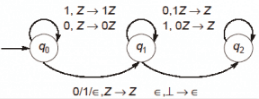Q. Which one of the following sequences must follow the string 101100 so that the overall string is accepted by the automaton?

Detailed Solution for Test: Context-Free Grammars & Push-Down Automata - Question 23

In q0 state for '1', a '1' is pushed and for a '0' a '0' is pushed. In q1 state, for a '0' a '1' is popped and for a '1' a '0' is popped. So, the given PDA is accepting all strings of of the form x0x'r or x1x'r or xx'r, where x'r is the reverse of the 1's complement of x. The given string 101100 has 6 letters and we are given 5 letter strings. So, x0 is done, with x = 10110. So, x'r = (01001)r = 10010. Hence option B is correct.

Test: Context-Free Grammars & Push-Down Automata - Question 24

Which of the following languages are context-free?

L1 = {ambnanbm ⎪ m, n ≥ 1}
L2 = {ambnambn ⎪ m, n ≥ 1}
L3 = {ambn ⎪ m = 2n + 1}

Detailed Solution for Test: Context-Free Grammars & Push-Down Automata - Question 24

We can build a push down automata for L1 and L3, but can not build push down automata for L@. Note that a PDA can uses a stack. L1 and L3 can be identified using a single stack, but L2 can't be.

Test: Context-Free Grammars & Push-Down Automata - Question 25

Which one of the following statements is FALSE?

Detailed Solution for Test: Context-Free Grammars & Push-Down Automata - Question 25

A) For real-world programming languages, the reference CFG is often ambiguous, due to issues such as the dangling else problem. //Wikipedia

B) A string is ambiguous if it has two distinct parse trees;The grammar is unambiguous,if a string has distinct parse trees.

C) Deterministic pushdown automata can recognize all deterministic context-free languages while nondeterministic ones can recognize all context-free languages

Therefore it's FALSE

D)Properties of Regular Language:

• The set of regular languages over an alphabet ∑ is closed under operations union, concatenation and Kleene star.
• Finite languages are regular

Test: Context-Free Grammars & Push-Down Automata - Question 26

If we use internal data forwarding to speed up the performance of a CPU (R1, R2 and R3 are registers and M is a memory reference), then the sequence of operations.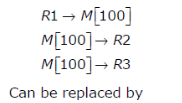Detailed Solution for Test: Context-Free Grammars & Push-Down Automata - Question 26

• Data Forwarding : In figure(2),ADD and SUB instructions have data dependency due to R1 registers,2nd and 3rd instructions read the register R1 value at ID stage but 1st instruction updates the value of R1 after WB stage .So 2nd SUB instruction is stalled for next two cycles to get updated value of R1 register.

• Internal data forwarding is a mechanism to reduces the stalls due to data dependency, it uses hardware technique to forward the result of interstage buffer register (IBR) to next instruction's buffer register. As soon as result is available after ALU operation (in 1st instruction), result is transferred as input to ALU unit, then updated value of R1 gets available after ALU operation (otherwise it is available after WB satge),so no stalls are there.

• The ALU result from the EX/MEM register is always fed back to the ALU input latches. If the forwarding hardware detects that the previous ALU operation has written the register corresponding to the source for the current ALU operation, con- trol logic selects the forwarded result as the ALU input rather than the value read from the register file.

• As given in Ques, It is straight from question,register R1 value is copied(or better say loaded) to memory location M then M's value is stored to registers R2 and R3 .Options A,B and C are wrong since they do not produce same result as desired.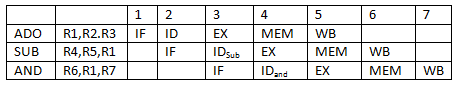• Let's suppose register R1,R2,R3 and memory reference M have initial values 10,20,30 and 40 respectively then after the execution of sequence of operation,registers R2,R3 and memory references M have values 10,10 and 10 respectively.

• In option A ,after the execution of operations,registers R2,R3 and memory references M have values 20,10 and 20 respectively. In option B, registers R2 , R3 and memory references M have values 10,10 and 40 respectively and option C ,reg- isters R2,R3 and memory references M have values 20,20 and 10 respectively . But option D produces ,all registers and memory reference have value same value 10 as desired , so option (D) is correct only.

Test: Context-Free Grammars & Push-Down Automata - Question 27

Consider the following context-free grammars: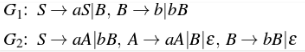Detailed Solution for Test: Context-Free Grammars & Push-Down Automata - Question 27

In G1, there will be atleast 1 b becase S->B and B->b. But no of A’s can be 0 as well and no of A and B are independent. In G2, either we can take S->aA or S->bB. So it must have atleast 1 a or 1 b. So option D is correct.

Test: Context-Free Grammars & Push-Down Automata - Question 28

Consider the pushdown automaton (PDA) below which runs over the input alphabet (a, b, c). It has the stack alphabet {Z0, X} where Z0 is the bottom-of-stack marker. The set of states of the PDA is (s, t, u, f} where s is the start state and f is the final state. The PDA accepts by final state. The transitions of the PDA given below are depicted in a standard manner. For example, the transition (s, b, X) → (t, XZ0) means that if the PDA is in state s and the symbol on the top of the stack is X, then it can read b from the input and move to state t after popping the top of stack and pushing the symbols Z0 and X (in that order) on the stack.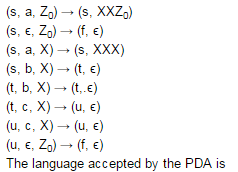Test: Context-Free Grammars & Push-Down Automata - Question 29

Consider the following languages.

L1 = {ai bj ck | i = j, k ≥ 1}
L1 = {ai bj | j = 2i, i ≥ 0}

Q. Which of the following is true?

Detailed Solution for Test: Context-Free Grammars & Push-Down Automata - Question 29

A: Both L1 and L2 are CFL
B: L1 ∩ L2 = ∅ is true
L1is not regular => true
=> B is true
C: L1 ∪ L2 is not a CFL nut L2 is CFL is closed under Union
=> False
D: Both L1 and L2 accepted by DPDA

Test: Context-Free Grammars & Push-Down Automata - Question 30

Consider a CFG with the following productions. S → AA | B A → 0A | A0 | 1 B → 0B00 | 1 S is the start symbol, A and B are non-terminals and 0 and 1 are the terminals. The language generated by this grammar is

Detailed Solution for Test: Context-Free Grammars & Push-Down Automata - Question 30

A− > 0A|A0|1 This production rule individually produces a CFL of the form {0i 10j |i, j ≥ 0} B− > 0B00|1 This production rule individually produces a CFL of the form {0n102n |n ≥ 0} S− > AA|B This production rule concatenates A’s CFL with itself and unions it with B’s CFL. Hence, CFL accepted by the given CFG will be {0n102n|n ≥ 0} ∪ {0i 10j 10k |i, j, k ≥ 0} According to our derivation, as none of the given CFL matches to our derived CFL, correct option should be (E) None of the above.

## GATE Computer Science Engineering(CSE) 2023 Mock Test Series

150 docs|215 tests
Information about Test: Context-Free Grammars & Push-Down Automata Page
In this test you can find the Exam questions for Test: Context-Free Grammars & Push-Down Automata solved & explained in the simplest way possible. Besides giving Questions and answers for Test: Context-Free Grammars & Push-Down Automata , EduRev gives you an ample number of Online tests for practice

## GATE Computer Science Engineering(CSE) 2023 Mock Test Series

150 docs|215 tests(Scan QR code)